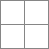# 4I Layout Pattern With Equal Sized Squares

How do I go about making a 4I layout pattern but with equal sized squares? I know I could just create a 2E layout and attach a 2U layout to each one, however this would require me to modify quite a bit of code to accommodate it, and I’d like to avoid doing this unless I must. Many thanks in advance.Here you can find the list of all the available patterns:
docs.dhtmlx.com/layout__patterns.html
In your case you may only use the combination of the 2E and 2U layouts.

I’m trying to see if it’s possible to create such a layout without having to nest a layout in one of the cells as to save a lot of time. I’ve been messing around with custom patterns and wondered if the following would be correct

``````dhtmlXLayoutObject.prototype.tplData["4B"] = '
<layout>
<autosize hor="b;d" ver="c;d" rows="2" cols="2"/>
<table data="a,b;c,d"/>
<row>
<cell obj="a" wh="2,2" resize="hor" neighbors="a;b"/>
<cell sep="ver" left="a" right="b" dblclick="a"/>
<cell obj="b" wh="2,2" resize="hor" neighbors="a;b"/>
</row>
<row sep="true">
<cell sep="hor" top="a,b" bottom="c,d" dblclick="a" colspan="3"/>
</row>
<row>
<cell obj="c" wh="2,2" resize="hor" neighbors="c;d"/>
<cell sep="ver" left="c" right="d" dblclick="c"/>
<cell obj="d" wh="2,2" resize="hor" neighbors="c;d"/>
</row>
</layout>
';
dhtmlXLayoutObject.prototype._availAutoSize["4B_hor"]=["a;c","b;d"];
dhtmlXLayoutObject.prototype._availAutoSize["4B_ver"]=["a;b","c;d"];``````